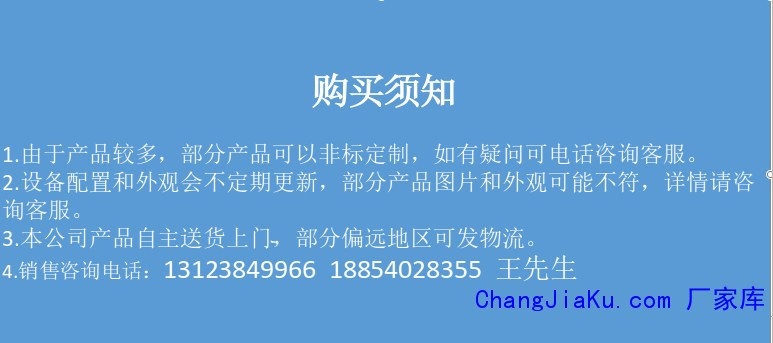客服热线：133-4342-757513123849966•••# 德迅DX-20KN 电子拉力试验机 拉力试验机 剥离试验机 拉力试验机

• 面议

CJK-1487

13123849966 057456215827
• 发货地  浙江
• 供货总量  99 台

• 暂无产品目录
 有效期至长期有效 更新时间2020-07-11 04:56 访问次数844

rr

r

r
r

r

r
r

r

r 使用领域：r

r

r 该产品广泛用于金属、非金属材料的拉、压、弯剪等力学性能试验。配以种类繁多的附具，还可用于型材和构件的力学性能试验。在试样变形大，试验速度快的绳、带、丝、橡胶、塑料等材料试验领域，同样具有非常广泛的应用前景。适用于质量监督、教学科研、航空航天、钢铁冶金、汽车、建工建材等试验领域。r

r

r 依据标准：r

r

r 满足标准GB/T228.1-2010《金属材料 室温拉伸试验方法》、GB/T7314-2005《金属压缩试验方法》的要求，符合GB、ISO、ASTM、DIN等多种标准的数据处理。可以符合用户的要求及提供的标准。r

r

r 四.外观照片：（请参考样本，以实物为准）r

r

r
r

r

r 重点描述：r

r

r 1.主机：该机采用双空间门式结构，上空间拉伸，下空间压缩，弯曲。横梁无级升降。传动部分采用圆弧同步齿形带，丝杠副传动，传动平稳，噪音低。特别设计的同步齿形带减速系统和精密滚珠丝杠副带动试验机的移动横梁运动，实现了无间隙传动。r

r

r 2.附具：r

r

r 标准配置：楔型拉伸附具、压缩附具各一套。r

r

r 3.电气测控系统：r

r

r （1）采用交流伺服系统和伺服电机，性能稳定、可靠，具有过流、过压、超速、过载等保护装置。r

r

r （2）具有过载、过流、过压、位移上下限位和紧急停止等保护功能。r

r

r （3）内置式控制器，保证了该试验机可以实现横梁位移等参量的控制，可实现等速位移。。r

r

r （4）试验结束时，可手动或自动高速返回试验初始位置。r

r

r （5）实现了真正意义上的物理调零、增益调整及试验力测量的自动换档、调零、标定和存盘，无任何模拟调节环节，控制电路高度集成化。r

r

r （6）电气控制线路参照国际标准，符合试验机电气标准，抗干扰能力强，保证了控制器的稳定性，实验数据准确性。r

r

r （7）具有网络接口，可进行数据的传输、存储、打印记录和网络传输打印，可与企业内部局域网或Internet网连接。r

r

r 4. 软件主要功能特点描述r

r

r 该测控软件用于微机控制电子试验机进行各种金属及非金属（如，人造板等）的试验，按照相应标准完成实时测量与显示、实时控制及数据处理、结果输出等各种功能。r

r

r (1)分权限管理，不同级别的操作者有不同的操作权限，可操作的菜单等内容也不同，既使普通操作者操作简单、方便、快捷，又有效的保护了系统；r

r

r (2)实时测量与显示试验力及峰值、位移、变形等各信号；实现了Win2000、WinXP等NT模式平台下的实时采集与控制；并实现了定时，高速采样；r

r

r (3)实现了负荷-变形，负荷-位移等多种试验曲线的实时屏幕显示，可随时切换观察，曲线的放大与缩小非常方便；r

r

r (4)试验参数的计算机存储、设定、加载等功能，调零、标定等操作都从软件上进行，各参数可方便的进行存储和调入，从而使一台主机带多个传感器时可以方便的切换，且没有数目限制；r

r

r (5)支持等速位移控制方式；并在操作者调试参数过程中给出标准参考曲线，从而使用户实际观察到各参数对效果产生的影响。r

r

r  (6)采用人机交互方式分析数据。处理方法满足应用广泛的《GB/T 228-2010金属材料室温拉伸试验方法》要求，可自动计算弹性模量、屈服强度、规定非比例延伸强度等各种性能参数，也可人工干预分析过程，提高分析的准确度；也可以根据用户提供的标准进行其他的数据处理。r

r

r (7)试验数据以文本文件存贮，以方便用户查询，以及利用任何通用商业报表、字处理软件对试验数据进行再处理，同时方便联网传递数据；r

r

r (8)可记录、保存试验全过程的数据曲线，并具有演示功能，实现试验曲线再现。还可以进行曲线叠加对比，便于对比分析；r

r

r (9)可按用户要求格式打印试验报告。用户可以自己选择报告输出基本信息和试验结果及试验曲线的内容，满足各种需要；r

r

r (10)实现了试验力和变形的数字调零、自动标定，方便了操作，提高了机器的可靠性。各种参数系统设置以文件形式存贮，便于保存和恢复；r

r

r (11)可应用于Win98、Win2000、WinXP等多种操作系统。试验过程控制、横梁移动速度的改变、参数输入等操作可全部用键盘、鼠标完成，使用方便快捷；r

r

r (12)可自动识别并支持外部点动控制，使装夹试样时很方便；r

r

r (13)具备过载保护自动停机功能，并可以自动判断试样断裂，自动停机。根据用户要求不同，上述软件功能会有增减或调整改变。r

r

r 5. 软件及软件操作界面：r

r

r （1）软件可以在Windows98/2000/XP，用户界面呈现与Windows风格一致的中文窗口系统。r

r

r
r

r

r (2)控制模式。r

r

r
r

r

r （3）报表编辑：r

r

r
r

r

r
r

r

r (4) 试验方法种类多,可选择r

r

r
r

r

r （5）软件设有三级管理权限，分别由各自的口令登录，进一步保证了软件安全使用。r

r

r
r

r

r 六.技术指标：r

rr r r r r r r r r r r r r r r r r r r r r r r r r r r r r r r r r r r r r r r r r r r r r r r r r r r r r r r r r r r r r r r r r r r r r r r r r r r r r r r r r r r r r r r r r r r r r
 r r 最大试验力（kN） r r r r 10 r r r r   r r r r 精度等级 r r r r 0.5级 r r r r   r r r r 试验力测量范围 r r r r r r r r   r r r r 试验力示值相对误差 r r r r ＜±0.5% r r r r   r r r r 试验力分辨率 r r r r 最大试验力的1/300000 r r r r   r r r r 变形测量范围 r r r r r r r r   r r r r 变形示值相对误差 r r r r ＜±0.5% r r r r   r r r r 变形分辨率 r r r r 最大变形量1/300000 r r r r   r r r r 位移示值相对误差 r r r r ＜±1% r r r r   r r r r 位移分辨力(mm) r r r r 0.001 r r r r   r r r r 速度范围 r r r r 0.01mm/min ~ 500mm/min r r r r 无级调速 r r r r 拉伸行程（mm） r r r r 800 r r r r   r r r r 压缩空间（mm） r r r r 800 r r r r   r r r r 试验宽度（mm） r r r r 400 r r r r   r r r r 可配夹具 r r r r 拉伸、压缩、弯曲、剪切、剥离、撕裂等 r r r r 主机外形尺寸（mm） r r r r 770*540*1730 r r r r   r r r r 伺服电机功率（kW） r r r r 0.75 r r r r   r r r r 总重量（kg） r r r r 350 r r r r   r r
rr

r 七.系统配置：r

rr r r r r r r r r r r r r r r r r r r r r r r r r r r r r r r r r r r r r r r r r r r r r r r r r r r r r r r r r r r r r r r r r r r r r r r r r r r
 r r 序号 r r r r 项目名称 r r r r 生产厂家及规格 r r r r 数量 r r r r 1 r r r r 主机框架 r r r r 铝合金 r r r r 1台 r r r r 2 r r r r 传动系统 r r r r 海德伺服系统 r r r r 1套 r r r r 3 r r r r 滚珠丝杠副 r r r r 冷拔钢轧制 r r r r 2套 r r r r 4 r r r r 负荷传感器 r r r r 美国世铨 r r r r 2只 r r r r 5 r r r r 计算机 r r r r 设备专用 r r r r 1台 r r r r 6 r r r r 打印机 r r r r 喷墨打印机 r r r r 1台 r r r r 7 r r r r 光电编码器 r r r r 2000线 r r r r 1个 r r r r 8 r r r r 软件及控制系统 r r r r ----- r r r r 1套 r r r r 9 r r r r 随机工具、随机文件 r r r r 使用说明书，安装手册等 r r r r 1套 r r r r 10 r r r r 标配附具 r r r r 1. 楔型拉伸附具 r r r r  平钳口：0-7 r r r r 2付 r r r r 2. 压缩附具 r r r r 压盘直径：φ r r r r 1套 r r
r

r
r

## 德迅 电子拉力试验机DWAW-300KN 液压式拉力r rrrrrrrrr主机为四立柱、两丝杠、油缸下置式，拉伸空间位于主机的上方，压缩、弯曲试验空间位于主机下横梁和工作台之间。测控系统采用全数字多通道闭环测控系统，具有三闭环功能，即可以进行应力、应变、位移闭环等控制方式，并可以进行无冲击切换，其应力速度和应变速率完全符合国标GB/T228.1-2010等其它金属拉伸试验标准要求。rrrrrrrrr二、传动系统rrr下横梁升降采用电机经减速器、链传动机构、丝杠副传动，实现拉伸、压缩空

## 数显扭力试验机 金属扭力试验机 弹簧扭力试## 德迅 电子拉力试验机DX-50KN 拉力试验机 压## 德迅 电子拉力试验机DX-100KN 拉力试验机## 自动疲劳试验机 波纹管油缸气弹簧疲劳试验## 德迅 DX-20000KN 大型剪压试验机 剪压试验## 德迅 WAW-600KN 拉力试验机 压力试验机 剥## 德迅 电子拉力试验机WAW-600KN 液压式拉力## 德迅 电子拉力试验机 DX-100KN 拉力试验机r rrrrrrrrr使用领域：rrr该产品广泛用于金属、非金属材料的拉、压、弯剪等力学性能试验。配以种类繁多的附具，还可用于型材和构件的力学性能试验。在试样变形大，试验速度快的绳、带、丝、橡胶、塑料等材料试验领域，同样具有非常广泛的应用前景。适用于质量监督、教学科研、航空航天、钢铁冶金、汽车、建工建材等试验领域。rrr依据标准：rrr满足标准GB/T228.1-2010《金属材料室温拉伸试验方法》、GB/T7314-2005《金属压缩试验方

## 德迅 电子拉力试验机DX-200KN 拉力试验机r rrrrrrrrr使用领域：rrr该产品广泛用于金属、非金属材料的拉、压、弯剪等力学性能试验。配以种类繁多的附具，还可用于型材和构件的力学性能试验。在试样变形大，试验速度快的绳、带、丝、橡胶、塑料等材料试验领域，同样具有非常广泛的应用前景。适用于质量监督、教学科研、航空航天、钢铁冶金、汽车、建工建材等试验领域。rrr依据标准：rrr满足标准GB/T228.1-2010《金属材料室温拉伸试验方法》、GB/T7314-2005《金属压缩试验方